Complex numbers

This free course is available to start right now. Review the full course description and key learning outcomes and create an account and enrol if you want a free statement of participation.

Free course

# 3.2 Relationship between complex numbers and points in the plane

We have seen in Section 2.2 that the complex number system is obtained by defining arithmetic operations on the set R × R. We also know that elements of R × R can be represented as points in a plane. It seems reasonable to ask what insight can be obtained by representing complex numbers as points of the plane.

One obvious way of making the representation is to use Cartesian coordinates. Then the number x + iy is represented by the point with coordinates (x, y). Thus if a point P represents a complex number z then its x coordinate is the real part of z and its y coordinate is the imaginary part of z (hence the choice of notation z = x + iy made in Section 2.2).

The horizontal axis is called the real axis and the vertical axis is called the imaginary axis.

This way of representing complex numbers by points in the plane is used so often that we shall use phrases such as “the point x + iy” and “the point z” as convenient shorthands for “the point representing z” and we shall speak of the complex plane. Figure 1 shows several points in the complex plane.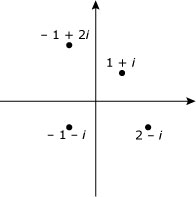Figure 1

As we saw in SAQ 11 in Section 2.4 (and it is fairly obvious anyway), the geometric representation can be taken a stage further. Complex numbers also add in the same way as number pairs regarded as vectors and so they add according to the parallelogram law (Figure 2).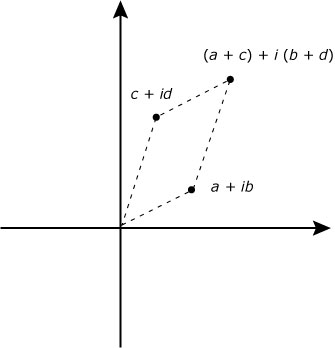Figure 2

Now points in the plane can also be represented by polar coordinates and this suggests another way of specifying a complex number.

If you have forgotten about polar coordinates, a short revision will not come amiss. When we use polar coordinates – instead of specifying a point in a plane by the distance from two lines, as in Cartesian coordinates – we specify a point P in the plane by its distance (r) from a fixed point O (the origin) and the angle (θ) that OP makes with a fixed half-line (the initial line) which has one end at O (Figure 3). (At present we consider only points P different from O.)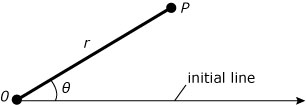Figure 3

As is usual in mathematics, the angle θ is measured in an anticlockwise direction from the initial line (Figure 4.).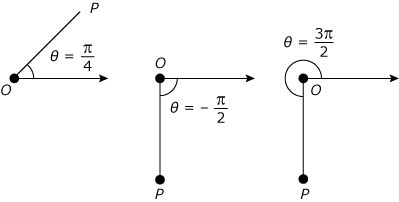Figure 4

One snag that does arise with polar coordinates is that they do not give a one-one correspondence with the points of the plane. Thus a point with polar coordinates (r, θ) also has polar coordinates (r, θ + 2k) where k is any integer. But polar coordinates (r, θ) do specify a unique complex number, and from the relationship between polar coordinates and Cartesian coordinates we know that this number is r cos θ + ir sin θ (Figure 5). So whether we use polar coordinates or Cartesian coordinates, a point in the plane specifies a unique complex number.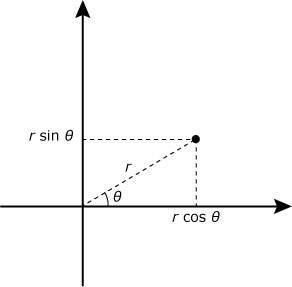Figure 5

It is often useful to represent a complex number in terms of the polar coordinates of the corresponding point as in the following definition.

## Definition

A polar form of the non-zero complex number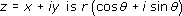where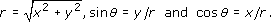The positive number r is called the modulus of z and θ is an argument of z. We denote the modulus of z by |z|, and so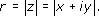For the special case of z = 0, we define |z| = 0, but we do not need to define what is meant by an argument of 0.

As we know, there will be some difficulty in specifying arguments. This is manifested by the fact that the relations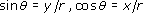specify θ only to within an integer multiple of 2. (Notice that the equation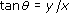is even less specific.)

Given any non-zero complex number, z, an argument can be any element from a whole set of numbers. For example, an argument of 1 + i can be any element from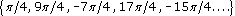. Nevertheless, any one element from this set is sufficient to identify the set and whatever the number z(≠0) there is only one of its arguments in the interval (−,].

## Definition

If θ is an argument of z and θ(−].θ is the principal value of the argument of z, and we write θ = Arg z. (Note the capital A.)

Thus, for example,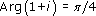(Figure 6).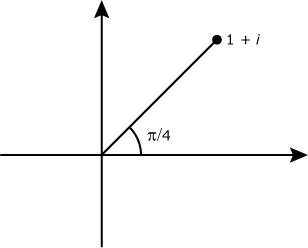Figure 6

It would have been just as reasonable to have chosen the interval [0.2) or (0.2] or [−,) for Arg z, but we must settle for one option – we happen to have chosen (−].

The polar form of a complex number can be very convenient and so we investigate now the effect of the arithmetic operations on the polar forms of complex numbers.

Addition and subtraction of complex numbers given in polar form is not a pleasant affair if the answers are also required to be in polar form. If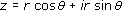and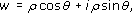then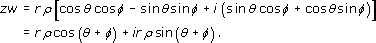and that is about as far as you can get unless you are a wizard at trigonometry. (The polar form would be p cos ψ + ip sin ψ where p and ψ are expressions in r, ρ, θ, and φ.) Of course, in any particular case you can work out the polar form of the answer, but the general result is not worth working out, let alone remembering.

On the other hand, multiplication is easy becauseThus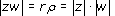and an argument of zw can be obtained just by adding arguments of z and w. If we want to work with principal values, there is one minor difficulty: even if θ and φ are in (−,], when we add θ and φ we might well get an answer outside (−,]. So if we want to work with principal values we shall have to make sure to get the answer back into (−,]. Thus although Arg z + Arg w is an argument of zw we may have to add or subtract 2to obtain Arg(zw).

One possible snag that may have occurred to you is this: if θ and φ are arguments of z and w respectively, is θ + φ an argument of zw whatever θ and φ we choose? Well, the relation where θ1 is related to θ2 if θ1 = θ2 + 2k, k an integer, is an equivalence relation on R and all the arguments of a complex number will constitute an equivalence class. We are asking whether we can combine equivalence classes (add arguments), in other words whether the equivalence relation is compatible with addition. Can we write

{arguments of z} + {arguments of w} = {arguments of zw}?

We can, of course, because if θ1 and θ2 are in the same equivalence class and φ1 and φ2 are in the same equivalence class, there are integers k and m such that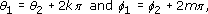and so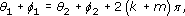that is, θ1 + φ1 is in the same equivalence class as θ2 + φ2.

We can also show that if α is a fixed argument of zw then we can choose an argument of z, θ say, and an argument of w, φ say, such that θ + φ = α.

Let α = Arg zw + 2n, where n is a fixed integer. Certainly,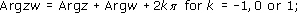and so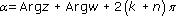So choose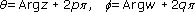where p + q = k + n.

We define division of complex numbers (as for real numbers) as the inverse of multiplication, and so it is no surprise that polar forms are also very suitable for division. If z−l is the number such that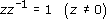then we define w ÷ z by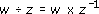As for real numbers, we write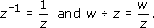We now ask how to calculate l/z when z(≠0) is given. If θ is an argument of z and φ an argument of 1/z then θ + φ is an argument of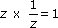. Since 0 is an argument of 1, we must be able to choose φ so that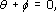and so −θ is an argument of 1/z. In fact, unless z is a negative real number, we can use principal values without trouble: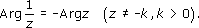(Note that a statement like k > 0 implies that k is real.)

If z = −k, k > 0, then Arg z =and Arg 1/z =.

What about |1/z|? We know that |1| = 1, and so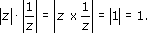Thus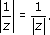We can now interpret division quite easily in terms of polar forms: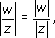and if θ and φ are arguments of z and w respectively, then θφ is an argument of w/z. More concisely,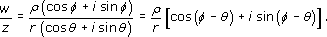To summarise: each of the arithmetic operations on C has a geometric interpretation. If z = x + iy is any complex number, corresponding to a point P, and if w = u + iv = p(cos φ +  sinφ) then:

1. the effect of adding w to z is to translate P to the point (x + u, y + v); the effect of subtracting w from z is to translate P to the point (xu, yv);

2. the effect of multiplying z by w is to rotate OP through the angle φ and “stretchOP by a factor ρ;

the effect of dividing z by w is to rotate OP through the angle −φ and “stretchOP by a factor 1/ρ.

The fact that when multiplying complex numbers their arguments are added suggests an interesting relationship between numbers z and w for which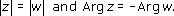Multiplying these two numbers gives a number with a zero argument – a real number (Figure 7). What is more, the modulus of this number is |z| · |w| and this is just |z|2. In polar form we see that if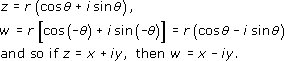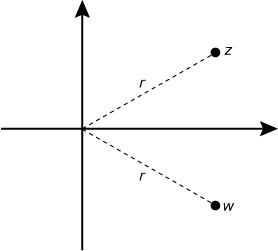Figure 7

The relationship between z and w is important and leads us to the following definition.

## Definition

If z = x + iy, the number xiy is the conjugate of z, and we write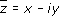(Note that many authors write z* for.)

We have just seen thathas the important property that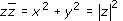.

This result is useful for dealing with quotients in Cartesian form, for an expression like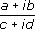could be simplified without resorting to polar form if we could make the denominator real. We now know how to do that. Since (cid)/(cid) = 1 , we can write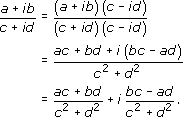The details of this expression are not worth remembering, but the technique is: multiply numerator and denominator by the conjugate of the denominator. It is often useful to remember that a denominator z becomes |z|2 after the process.

Perhaps the most impressive payoff from writing complex numbers in polar form arises in the problem of solving an equation of the form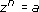where a is a given non-zero complex number. Now it is easy to deduce from our knowledge of multiplication of polar forms that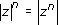and that if θ is an argument of z then nθ is an argument of zn. (See SAQ 21 in Section 3.4.) So if zn = a, z must be a number whose modulus is an nth root of a and which has an argument which is 1/n times an argument of a.

## Example 1

Find a value of z such that z4 = 16i.

### Solution

Since |16i| = 16, we have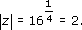An argument of 16i is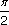, so an argument of z is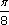, because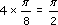. So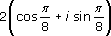is a possible value of z. But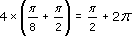and this is also an argument of a, so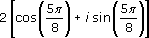is another possible value of z.

This example clearly suggests that we need a systematic method of finding all the solutions of the equation zn = a, a ≠ 0. We can see how to do this by considering the simpler problem of finding all the solutions of wn = 1. Since |1| = 1, we must have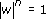and if φ is an argument of w, nφ must be a multiple of 2because 0 is an argument of 1. In other words, |w| = 1 and any of the values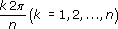can be an argument of w – each leading to a different value of w. (If k takes integer values beyond n, we get repetitions of the values of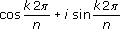that have already been obtained.) Thus in the set of complex numbers, the number 1 has n nth roots: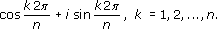The points representing the roots lie equally spaced around the unit circle. Figure 8 shows the roots for n = 8.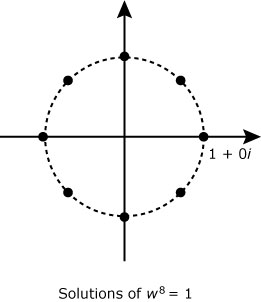Figure 8

Now let us get back to the original problem: zn = a, a ≠ 0. We do not seem to have any difficulty in finding one solution of this equation: the problem is how to find the whole set of solutions. Let us suppose we have one solution, z0, so that z0n = a. We now use the solution to the simplified problem, wn = 1. Suppose w is a solution of this problem, then (wz0)n = wnz0n = 1a = a, and so wz0 is a solution to the original problem. But there are n possibilities for w and so there are n possibilities for wz0 (one of which is z0 because one of the values of w is 1). So knowing the solutions of wn = 1 (which is easy) and just one solution of zn = a (which is not too bad) we can generate n solutions of zn = a. The question is: “Have we now got them all?”. It would be most surprising if we had not, but we still have to check – or rather you have to check, because that is SAQ 22 in Section 3.4.

In solving the equation zn = a in the set of complex numbers we are extending the notion of roots of a real number and so we make the following definition.

## Definition

The solutions of zn = a are called nth roots of a.

We have proved the following theorem (taking SAQ 22 of Section 3.4 for granted).

## Theorem 1

Every non-zero complex number has exactly n distinct nth roots.

We find the n nth roots of a ≠ 0 by using the following technique.

Technique

If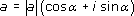then one solution of zn = a is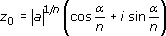and all the others are obtained by multiplying z0 in turn by each of the nth roots of wn = 1.

Now the effect of multiplying each of the n nth roots of 1 by z0 will be to multiply each of their moduli (all equal to 1) by |a|1/n and add α/n to each of their arguments. In other words, the nth roots of the complex number a are obtained from the nth roots of 1 by expanding (or contracting) the unit circle by |a|1/n and rotating by (Arg a)/n. The nth roots of a will be equally spaced around a circle of radius |a|1/n. The roots are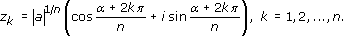Figure 9 illustrates this for n = 8 and |a| > 1.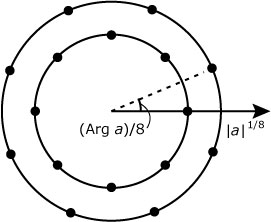Figure 9

## Example 2

To find the solutions of z4 = 1 + i, the fourth roots of 1 + i, we proceed as follows.

1.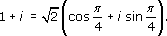2.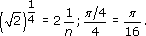3. Thus one root is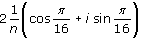and the others can be found because the four of them are equally spaced around the circle of radius(Figure 10).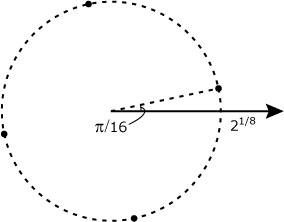Figure 10
M332_1

### Take your learning further

Making the decision to study can be a big step, which is why you'll want a trusted University. The Open University has 50 years’ experience delivering flexible learning and 170,000 students are studying with us right now. Take a look at all Open University courses.

If you are new to university level study, find out more about the types of qualifications we offer, including our entry level Access courses and Certificates.

Not ready for University study then browse over 900 free courses on OpenLearn and sign up to our newsletter to hear about new free courses as they are released.

Every year, thousands of students decide to study with The Open University. With over 120 qualifications, we’ve got the right course for you.

Request an Open University prospectus